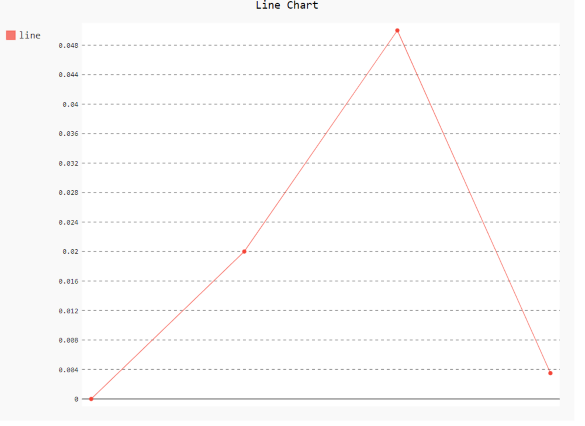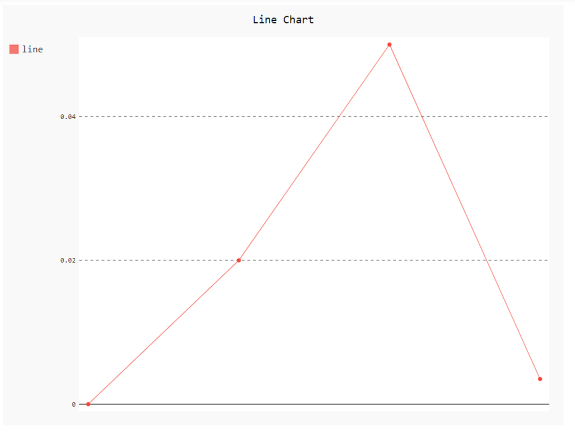GeeksforGeeks App
Open AppBrowser
Continue

# How to change number of scales in pygal?

Prerequisites:pygal

Pygal is a Python module that is mainly used to build SVG (Scalar Vector Graphics) graphs and charts. Pygal is a graphics and user interface library for Python that provides functionality commonly required in designing and science applications.

In this article, we will see how we can change the number of scales in pygal. A scale on the graph shows the way the numbers or pictures are used in data. The scale selected for a graph axis has a significant impact on how the audience interprets the message and is an important part of optimizing data visualization. Thus, a scale plays a crucial part in plotting graphs.

Approach

• Import required module.
• Create a chart object.
• Pass maximum/minimum number of scale.
• Label the graph.
• Display Graph.

Syntax:

• min_scale
• max_scale

You can specify the maximum/minimum number of scale graduation to generate with auto-scaling if possible.

Example 1:

## Python3

 `# importing pygal``import` `pygal``import` `numpy`` ` ` ` `# creating the chart object``# minimum number of scale``chart ``=` `pygal.Line(min_scale``=``40``)`` ` `# Random data``chart.add(``'line'``, [``0``, .``02``, .``05``, .``0035``])`` ` `# naming the title``chart.title ``=` `'Line Chart'`` ` `chart.render_to_png(``'aa.png'``)`

OutputExample 2:

## Python3

 `# importing pygal``import` `pygal``import` `numpy`` ` ` ` `# creating the chart object``# maximum number of scale``chart ``=` `pygal.Line(max_scale``=``3``)`` ` `# Random data``chart.add(``'line'``, [``0``, .``02``, .``05``, .``0035``])`` ` `# naming the title``chart.title ``=` `'Line Chart'`` ` `chart.render_to_png(``'aa.png'``)`

OutputMy Personal Notes arrow_drop_up